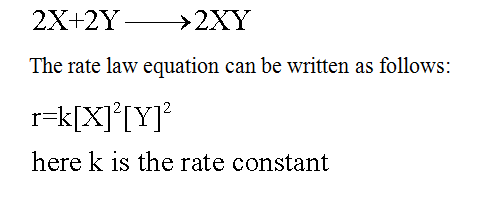# Consider this two-step mechanism for a reaction:NO2+Cl2=ClNO2+Cl   slowNO2+Cl=ClNO2    fastIdentify the intermediates in the mechanism and the predicted rate law based on the mechanism.

Question
11 views

Consider this two-step mechanism for a reaction:

NO2+Cl2=ClNO2+Cl   slow

NO2+Cl=ClNO2    fast

Identify the intermediates in the mechanism and the predicted rate law based on the mechanism.

check_circle

Step 1

The rate of a given reaction can be defined as the speed at which the conversion of reactants into the products takes place.

Step 2

The term rate law equation or rate equation for a given chemical reaction can be defined as the equation which is used to link the rate of the reaction with the pressures or the concentrations of reactants. For a given chemical equation, say,Step 3

The rate determining step for a given reaction mechanism can be defined as the slowest step in it and this step will determine the rate of the overall chemical reaction.

In the given multistep reaction, the rate of each step involved in the ...

### Want to see the full answer?

See Solution

#### Want to see this answer and more?

Solutions are written by subject experts who are available 24/7. Questions are typically answered within 1 hour.*

See Solution
*Response times may vary by subject and question.
Tagged in